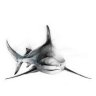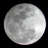# Colored Candles RSI based for ThinkorSwim#### horserider

##### Well-known member
VIP
Updated the study allowing user input of the High and low threshold values. User can now adjust those levels and the length input without having to modify the code.
Seems good at showing change of direction with length 2, exponential, 58, 38 . Play till you get what you want.

Code:
``````## RSI Modified for Charlie Baker, by Waylock on June 19, 2016
## Color Price Chart based on 40 -60 Level

Input Length = 16;
Input AverageType = AverageType.WILDERS;

Def Price = (High + Low + Close) / 6;
Def NetChgAvg = MovingAverage(AverageType, Price - Price, Length);
Def TotChgAvg = MovingAverage(AverageType, AbsValue(Price - Price), Length);
Def ChgRatio = if TotChgAvg != 0 then NetChgAvg / TotChgAvg else 0;
Def RSI = 50 * (ChgRatio + 1);
Def AvgExp = ExpAverage(RSI, 3);

input hv = 60;
input lv = 40;
AssignPriceColor(if AvgExp >hv then Color.GREEN else if AvgExp < lv then Color.RED else Color.YELLOW);``````

Old study is below.
Since people requested color candles. One of many color candles indicators. Play with settings to adjust to your preference or time frame. Shown is 5 min. Length 12.Code:
``````## RSI Modified for Charlie Baker, by Waylock on June 19, 2016
## Color Price Chart based on 40 -60 Level

Input Length = 16;
Input AverageType = AverageType.WILDERS;

Def Price = (High + Low + Close) / 3;
Def NetChgAvg = MovingAverage(AverageType, Price - Price, Length);
Def TotChgAvg = MovingAverage(AverageType, AbsValue(Price - Price), Length);
Def ChgRatio = if TotChgAvg != 0 then NetChgAvg / TotChgAvg else 0;
Def RSI = 50 * (ChgRatio + 1);
Def AvgExp = ExpAverage(RSI, 3);

AssignPriceColor(if AvgExp > 58 then Color.GREEN else if AvgExp < 38 then Color.RED else Color.YELLOW);``````

Last edited:
••jay2, Rojo Grande, thinky and 5 others#### mc01439

##### Well-known member
2019 Donor
VIP
Very Nice Job! Thanks for sharing.#### markos

##### Well-known member
VIP
@horserider Thanks for keeping the header intact. I appreciate it.

Colored Candles, Colored Bars, Colored Heiniken Ashley Bars, wow, I'm thirsty!Sometimes I amused myself!!

Last edited:
•Rojo Grande#### horserider

##### Well-known member
VIP
Updated this to allow user inputs. Details in first post.

•jay2 and BenTen#### Bidtheoffer

##### New member
Found this RSI on Tradeview with a very interesting plotting to it i was wondering if anyone can help me edit it so far i keep running into walls have taken rsi studies in order to modified and yet it has been failures, if anyone can help out that would be great and it will also show me were i'm failing, thank you.

Code:
``````//@version=3
study(title="Chop and explode", shorttitle="CAE")

src = close, len = input(14, minval=1, title="Length")
up = rma(max(change(src), 0), len)
down = rma(-min(change(src), 0), len)
rsi = down == 0 ? 100 : up == 0 ? 0 : 100 - (100 / (1 + up / down))

plot(rsi, color=black)

change1 = rsi > 60 ? blue :na
change2= rsi < 40 ? purple: na

p1 = plot(rsi, style=linebr, linewidth=3, color=change1)
p2 = plot(rsi, style=linebr, linewidth=3, color=change2)

band1 = hline(70)
band2 = hline(30)
band3 = hline (60)
band4 = hline (40)
midline= hline (50)
band5=hline(55)
band6=hline(45)
fill(band1, band3, color=green, transp=90)
fill(band3, band4, color=yellow, transp=90)
fill(band4, band2, color=red, transp=90)
fill (band5,band6, color=black, transp=50)

cond1 = rsi > 60 and close < rsi and close > 0 ? 1 : 0
barcolor(cond1 ? blue : na)

cond2 = rsi < 40 and close < rsi and close > 0 ? 1 : 0
barcolor(cond2 ? purple : na)

cond3 = rsi > 55 and close < rsi and close < 60 ? 1 : 0
barcolor(cond3 ? yellow : na)

cond4 = rsi > 45 and close < rsi and close  < 55 ? 1 : 0
barcolor(cond4 ? black : na)

cond5 = rsi > 40 and close < rsi and close  < 55 ? 1 : 0
barcolor(cond5 ? yellow : na)``````

Last edited:
D

#### DeusMecanicus

##### Member
I made a few modifications to horserider's original post to give this indicator a few more RSI zones. I've used it on the 5 min for a few scalps on pullbacks to VWAP (beware if there is a news catalyst though, sometimes it will keep pushing through!). Looking at the past daily chart some bottoms and tops really jump out at you. I also like it for pullbacks if the trend is intact. After the candle turns yellow and settles against a MA look for it to continue after changing back in the way of the trend.

Code:
``````## RSI Modified for Charlie Baker, by Waylock on June 19, 2016
## Color Price Chart based on 40 -60 Level
## Modified for more zones

Input Length = 7;
Input AverageType = AverageType.WILDERS;

Def Price = (High + Low + Close) / 6;
Def NetChgAvg = MovingAverage(AverageType, Price - Price, Length);
Def TotChgAvg = MovingAverage(AverageType, AbsValue(Price - Price), Length);
Def ChgRatio = if TotChgAvg != 0 then NetChgAvg / TotChgAvg else 0;
Def RSI = 50 * (ChgRatio + 1);
Def AvgExp = ExpAverage(RSI, 3);

input hv = 60;
input lv = 40;
input hvh = 80;
input lvl = 20;
input hvhh = 90;
input lvll = 10;
input hvhhh = 95;
input lvlll = 5;

AssignPriceColor(if AvgExp <lvlll then Color.BLACK else if AvgExp >hvhhh then Color.BLUE else if AvgExp <lvll then Color.DARK_GRAY else if AvgExp >hvhh then Color.CYAN else if AvgExp <lvl then Color.DARK_RED else if AvgExp >hvh then Color.DaRK_GREEN else if AvgExp >hv then Color.green else if AvgExp < lv then Color.red else Color.YELLOW);``````

•jay2 and BenTen
J

#### jay2

##### New member
AssignPriceColor(if AvgExp <lvlll then Color.BLACK else if AvgExp >hvhhh then Color.BLUE else if AvgExp <lvll then Color.DARK_GRAY else if AvgExp >hvhh then Color.CYAN else if AvgExp <lvl then Color.DARK_RED else if AvgExp >hvh then Color.DaRK_GREEN else if AvgExp >hv then Color.green else if AvgExp < lv then Color.red else Color.YELLOW);
Can you explain the meaning of the colored bars? like Dark Red, Dark Green, Cyan?

J

#### jay2

##### New member
nm.. put it to test today. reaching RSI highs (cyan / dark green) and lows (dark red)

A

#### ala

##### New member
HI, any one know how to convert this pine scrip into think scrip? i will be thankful.

Code:
``````src = close, len = input(14, minval=1, title="Length")
up = rma(max(change(src), 0), len)
down = rma(-min(change(src), 0), len)
rsi = down == 0 ? 100 : up == 0 ? 0 : 100 - (100 / (1 + up / down))

plot(rsi, color=black)``````Indicators 3